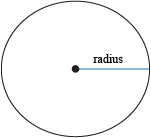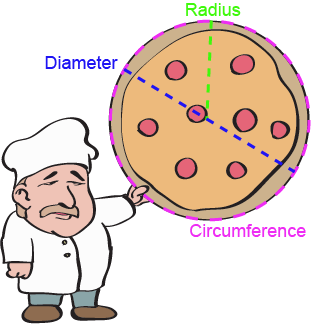## Area of Circles

So far, we've been looking at areas of triangles and rectangles and trapezoids. Shapes with straight edges and angles and corners. Well, it was fun while it lasted, but we're done with all that business. Time to get a little less square and a little more well-rounded.

We know what a circle is. We know what area is. Need we say more? Uh, probably.

A circle doesn't have any straight lines. It's just a never-ending curve that goes around and around. That being the case, there's no such thing as a base and height. They just don't exist for circles. But if they don't exist, how can we find the area of a circle? Nooo!

Okay, so it isn't that terrible. If we don't have a base and a height, what do we have to work with? As we know, a circle is described by its radius r, which is the distance from the center of the circle to its edge.As it turns out, the area of a circle can be given by the formula A = πr2, where r is the radius and π is the never-ending irrational number that we can estimate as 3.14. We wish it were key lime, but that might just be too much to hope for.

After all, pies are round, but pi r squared.

### Sample Problem

What is the area of a circle with a radius of 3 cm?

To find the area of a circle, we only need to know the radius. After that, just apply the formula for the area of a circle.

A = πr2
A = π(3 cm)2
A = 9π cm2 ≈ 28.3 cm2

### Sample Problem

A ship malfunction causes a circular oil spill that covers 4.52 square miles of the ocean. What is the length across the entire oil spill?

Even in unfortunate situations such as these, we can still use the formula A = πr2 when dealing with the area of a circle.

A = πr2
4.52 miles2 = πr2
r2 ≈ 1.44 miles2
r ≈ 1.2 miles

Since the radius is the distance from the edge to the center, we need to multiply it by 2 so that it reaches from one side of the oil spill to the other. The distance across the entire oil spill is 2 × 1.2 miles = 2.4 miles.

In addition to the radius, another term to describe a circle is the diameter d, which is twice the length of the radius. The diameter goes all the way across the circle, through its center, kind of like a belt. But you knew that.

The pizza guy from Inconvenient Pizzas, Inc. might ask you whether you want a 6-inch pizza (if you're hungry), a 12-inch pizza (if you're really hungry), or even an 18-inch pizza (if you're a hungry hungry hippo). These dimensions are the diameter of the pizza you're ordering. Even though you only have the diameter, you can still figure out the area. Though with Inconvenient Pizzas, you can't be sure what you order is what you get.

The perimeter of a circle, a.k.a. the circumference C, can also be used to find the area. In general, as long as we can find the radius of a circle r, we can find the area.We can convert diameter into radius (just divide d by 2 to get r) and circumference into radius (use the formula C = 2πr and isolate for r). Then we just have to use the formula A = πr2 to solve for area. Easier than a piece of π, right?

### Sample Problem

Aliens have arrived! You could've sworn you heard a weird humming during the night and some strange chatter outside. Sure enough, they've made a mark on your farm in the form of a crop circle with a diameter of exactly 6 kilometers. What is the area of the crop circle?

We know that the diameter of the circle is 6 km, which means the radius of the circle is d2 or 3 km. As long as we know the radius of a circle, we can find its area faster than the speed of light using our trusty area formula.

A = πr2
A = π(3 km)2
A = 9π ≈ 28.3 km2

The area of the crop circle with a diameter of 6 km is 9π, or about 28.3 km2.

We can also find the areas of portions of circles (called sectors) as long as we know central angle of the sector. For instance, a semicircle is a sector with a central angle of 180° (or π radians) and its area is half that of a circle with the same radius. We can generalize this for all sectors and come up with two formulas (depending on whether the central angle of the sector, θ, is in degrees or radians).

A = θ360° × πr2
A = θ × πr2